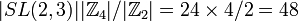# Central product of SL(2,3) and Z4

View a complete list of particular groups (this is a very huge list!)[SHOW MORE]

## Definition

This group is defined as the central product of special linear group:SL(2,3) and cyclic group:Z4 with a common central cyclic group:Z2 identified (i.e., center of special linear group:SL(2,3) is identified with Z2 in Z4).

## Arithmetic functions

Want to compare and contrast arithmetic function values with other groups of the same order? Check out groups of order 48#Arithmetic functions
Function Value Similar groups Explanation
order (number of elements, equivalently, cardinality or size of underlying set) 48 groups with same order product formula: The order of the central product is$|SL(2,3)||\mathbb{Z}_4|/|\mathbb{Z}_2| = 24 \times 4/2 = 48$.

## GAP implementation

### Group ID

This finite group has order 48 and has ID 33 among the groups of order 48 in GAP's SmallGroup library. For context, there are groups of order 48. It can thus be defined using GAP's SmallGroup function as:

SmallGroup(48,33)

For instance, we can use the following assignment in GAP to create the group and name it$G$:

gap> G := SmallGroup(48,33);

Conversely, to check whether a given group$G$ is in fact the group we want, we can use GAP's IdGroup function:

IdGroup(G) = [48,33]

or just do:

IdGroup(G)

to have GAP output the group ID, that we can then compare to what we want.